# 20个Pandas函数详解

08/10 23:29

Pandas是一个python数据分析库。它提供了许多函数和方法来加快数据分析过程。pandas之所以如此普遍，是因为它具有强大的功能，以及他简单的语法和灵活性。

``````import numpy as np
import pandas as pd
``````

### 1.query

``````values_1 = np.random.randint(10, size=10)
values_2 = np.random.randint(10, size=10)
years = np.arange(2010,2020)
groups = ['A','A','B','A','B','B','C','A','C','C']
df = pd.DataFrame({'group':groups, 'year':years, 'value_1':values_1, 'value_2':values_2})
df
``````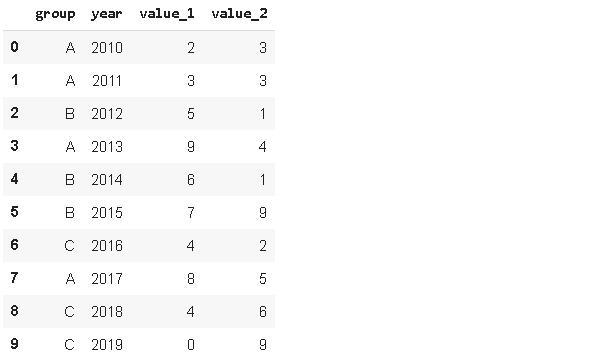``````df.query('value_1 < value_2')
``````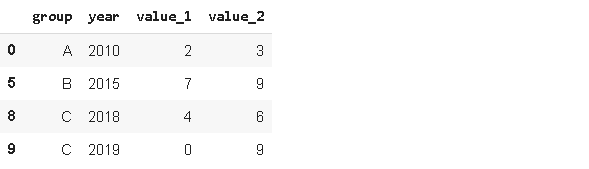### 2.insert

``````#新建列
new_col = np.random.randn(10)
#在位置2插入新列
df.insert(2, 'new_col', new_col)
df
``````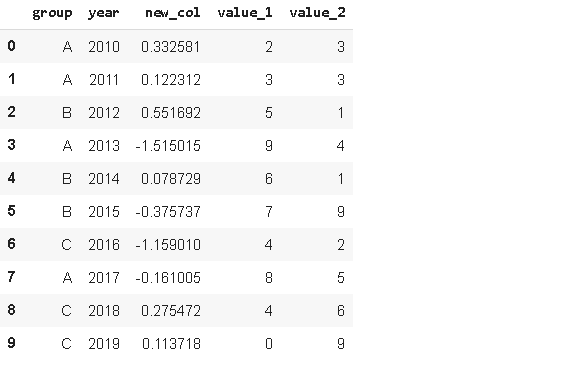### 3.Cumsum

``````df['cumsum_2'] = df[['value_2','group']].groupby('group').cumsum()
df
``````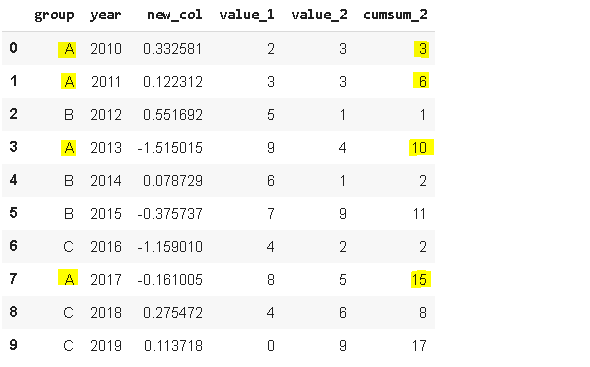### 4.Sample

Sample方法允许你从序列或数据帧中随机选择值。当我们想从一个分布中选择一个随机样本时，它很有用。

``````sample1 = df.sample(n=3)
sample1
````````````sample2 = df.sample(frac=0.5)
sample2
``````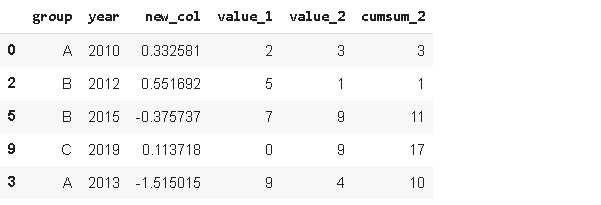### 5. Where

“Where”用于根据条件替换行或列中的值。默认的替换值是NaN，但是我们也可以指定替换的值。

``````df['new_col'].where(df['new_col'] > 0 , 0)
``````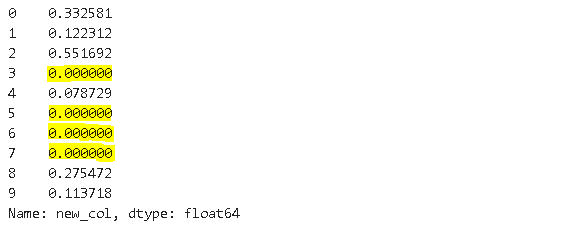“where”的工作方式是选择符合条件的值，其余值替换为指定值。

**where(df[‘new_col’]>0, 0)**选择“new_col”中大于0的所有值，其余值替换为0。因此，这里也可以视为掩码操作。

``````df['new_col'].where(df['new_col'] > 0 , 0)
np.where(df['new_col'] < 0, df['new_col'], 0)
``````

### 6.Isin

``````years = ['2010','2014','2017']
df[df.year.isin(years)]
``````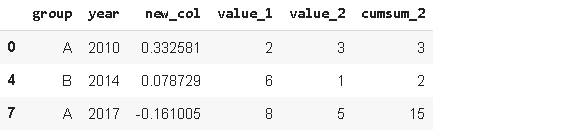### 7.Loc and iloc

Loc和iloc用于选择行和列。

• loc：按标签选择

• iloc：按位置选择

loc用于按标签选择数据。列的标签是列名。行标签要分情况，如果我们不分配任何特定的索引，pandas默认创建整数索引。iloc 按位置索引数据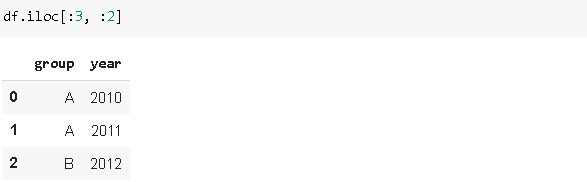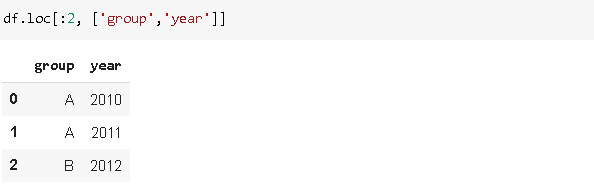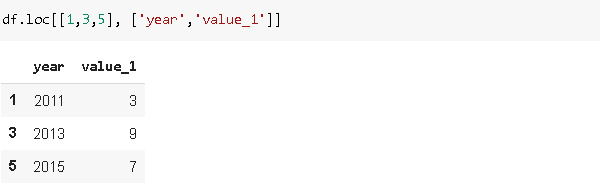### 8.Pct_change

``````df.value_1.pct_change()
``````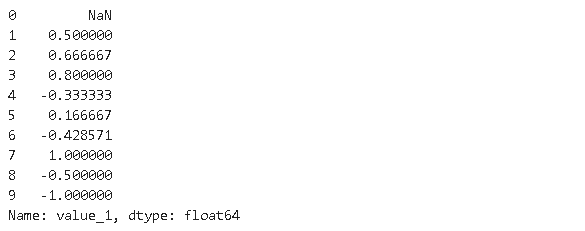### 9.Rank

Rank函数为值分配序。假设我们有一个包含[1,7,5,3]的序列s。分配给这些值的序为[1,4,3,2]。可以用这些序作排序操作

``````df['rank_1'] = df['value_1'].rank()
df
``````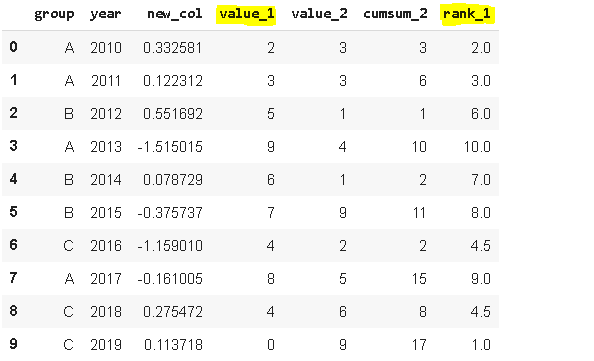### 10.Melt

Melt用于将宽数据帧转换为窄数据帧。我所说的wide是指具有大量列的数据帧。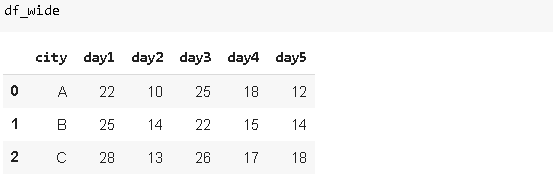``````df_wide.melt(id_vars=['city'])
``````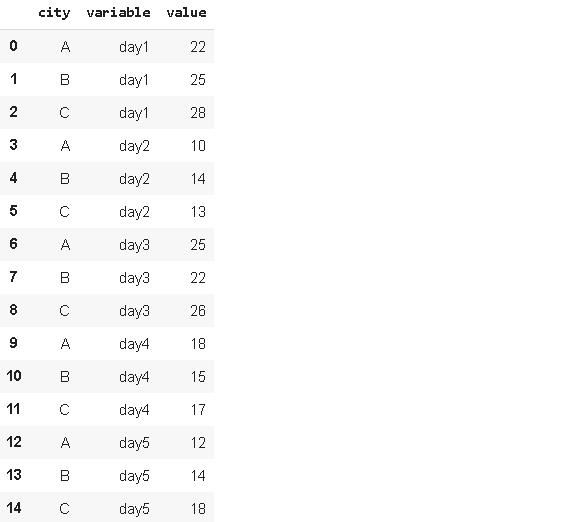### 11.Explode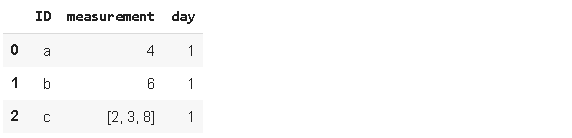``````df1.explode('measurement').reset_index(drop=True)
``````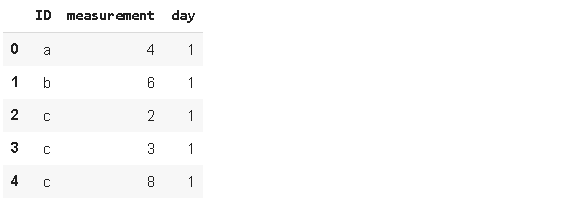### 12.Nunique

Nunique统计列或行上的唯一条目数。它在分类特征中非常有用，特别是在我们事先不知道类别数量的情况下。让我们看看我们的初始数据帧：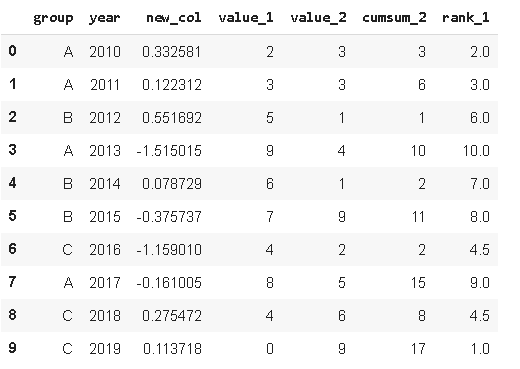``````df.year.nunique()
10
df.group.nunique()
3
``````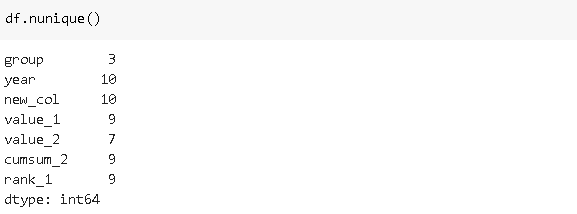### 13.lookup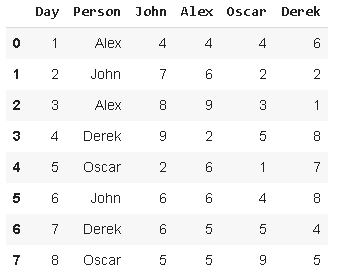``````df['Person_point'] = df.lookup(df.index, df['Person'])
df
``````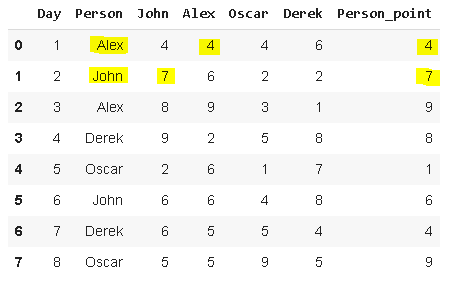### 14.Infer_objects

Pandas支持广泛的数据类型，其中之一就是object。对象包含文本或混合(数字和非数字)值。

infer_objects尝试为对象列推断更好的数据类型。考虑以下数据帧：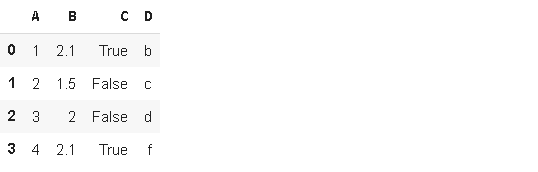``````df2.dtypes
A    object
B    object
C    object
D    object
dtype: object
``````

``````df2.infer_objects().dtypes
A      int64
B    float64
C       bool
D     object
dtype: object
``````

### 15.Memory_usage

Memory_usage返回每行使用的内存量(以字节为单位)。它非常有用，尤其是当我们处理大型数据帧时。考虑下面的数据帧，其中有一百万行。

``````df_large = pd.DataFrame({'A': np.random.randn(1000000),
'B': np.random.randint(100, size=1000000)})
df_large.shape
(1000000, 2)
``````

``````df_large.memory_usage()
Index        128
A        8000000
B        8000000
dtype: int64
``````

``````df_large.memory_usage().sum() / (1024**2)
15.2589111328125
``````

### 16.Describe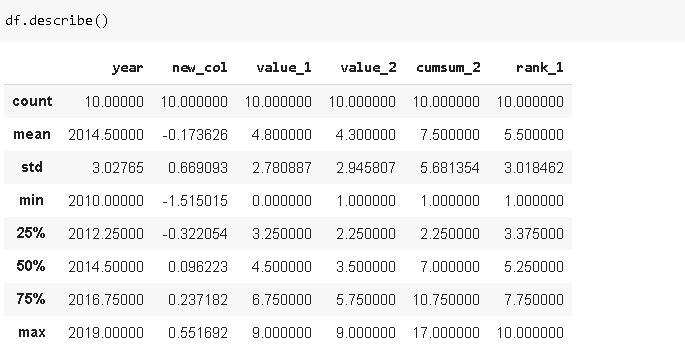### 17.Merge

Merge()根据共享列中的值组合数据帧。考虑以下两个数据帧。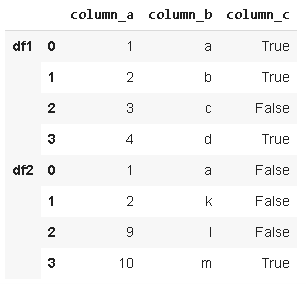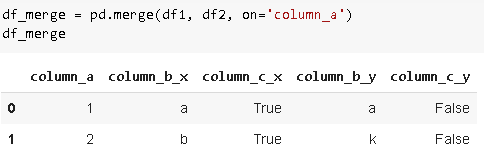df1和df2是基于column_a中的公共值进行合并的，merge函数的how参数允许以不同的方式组合数据帧。“内部”、“外部”、“左侧”、“右侧”的可能值。

• inner：仅在on参数指定的列中具有相同值的行（how参数的默认值)

• outer：所有行

• left：左数据帧中的所有行

• right：右数据帧中的所有行

### 18.Select_dtypes

Select_dtypes函数根据对数据类型设置的条件返回数据帧列的子集。它允许使用include和exlude参数包含或排除某些数据类型。

``````df.select_dtypes(include='int64')
``````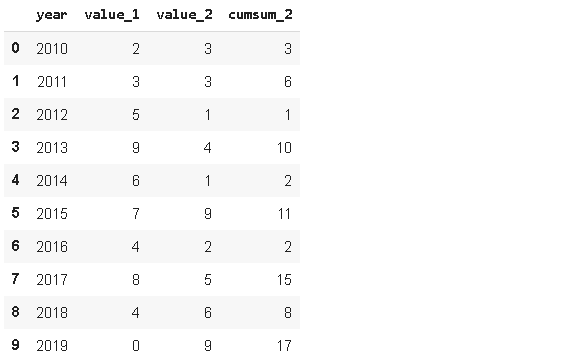``````df.select_dtypes(exclude='int64')
``````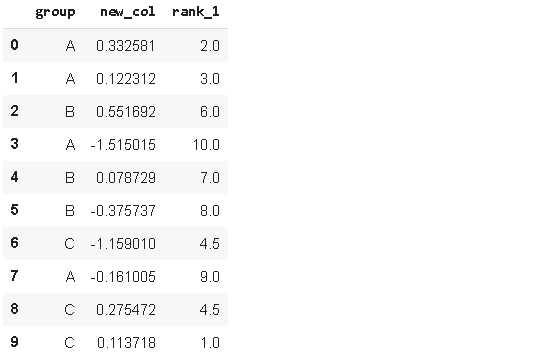### 19.replace

``````df.replace('A', 'A_1')
``````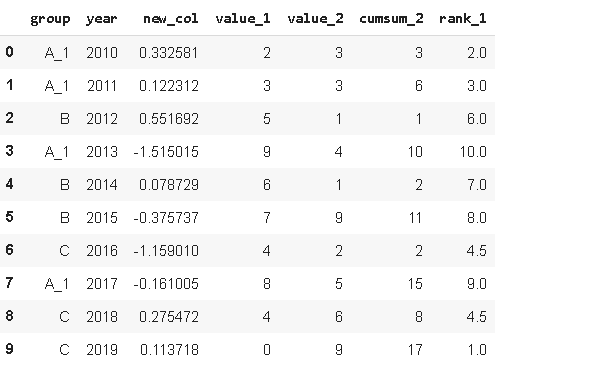``````df.replace({'A':'A_1', 'B':'B_1'})
``````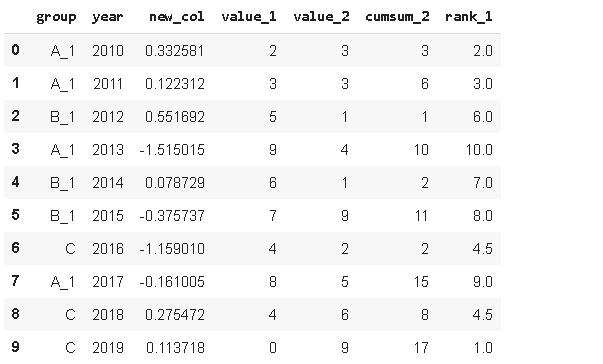### 20.Applymap

Applymap函数用于将函数应用于dataframe元素。

``````def color_negative_values(val):
color = 'red' if val < 0 else 'black'
return 'color: %s' % color
``````

``````df3.style.applymap(color_negative_values)
``````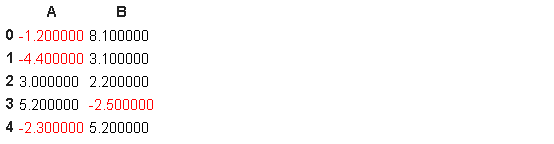sklearn机器学习中文官方文档： http://sklearn123.com/

0
0 收藏

### 作者的其它热门文章0 评论
0 收藏
0